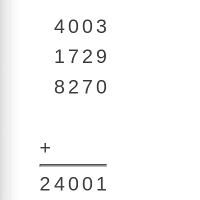A Magical Addition Trick

Here's a neat addition trick my uncle taught me

By

I must have been in about fourth or fifth grade. I was visiting my grandparents, when my uncle got out a pen and paper, saying he had a math trick to show me. He explained that by magic he could predict in advance what the result of an addition sum would be.

Now, I know it wasn't magic at all, but by the end of the trick, it certainly seemed magical. Here's how it worked.

First, he asked me to write down a four-digit number, like so.

 4 0 0 3

Then, he predicted the final result of the sum, and wrote it down.

 4 0 0 3 + 2 4 0 0 1

How on earth could he predict it? I wondered. Next, he explained there were still four more four-digit numbers to write in the sum, and we would take turns writing them, and I would start. So, I took the pen and wrote.

 4 0 0 3 1 7 2 9 + 2 4 0 0 1

He took the pen, and without hesitation, he filled in the next number.

 4 0 0 3 1 7 2 9 8 2 7 0 + 2 4 0 0 1

It was my turn to write. I took the pen.

 4 0 0 3 1 7 2 9 8 2 7 0 4 1 0 4 + 2 4 0 0 1

He smiled, and again with no hesitation, wrote the final number in the sum.

 4 0 0 3 1 7 2 9 8 2 7 0 4 1 0 4 + 5 8 9 5 2 4 0 0 1

He gave me back the pen, and said. There. Now you check the sum. Now checking such a sum is not an easy thing for a fourth-grader! A calculator would have helped. But sure enough, his predicted answer was correct! How did he do it? My uncle wasn't a genius mathematician, yet his claim of 'magic' didn't sound convincing to me! How did this mathematical magic work?

In the next few paragraphs, I'll explain how it works, so you can show the fifth-graders in your lives the same trick. Hopefully they'll be as impressed as I was when they see it done. Even more importantly, I hope that they'll learn what I did about the hidden secrets of arithmetic when you show them how it works.

First, explain the trick - that you will predict the outcome of an arithmetic sum. Ask the child to write down a four-digit number.

 2 7 8 1

Then, it's your turn to predict the answer. To get your prediction, subtract two from from the child's number, and put a two in front. In this case, that gives 2781 - 2 = 2779. So with a two in front, your prediction becomes:

 2 7 8 1 + 2 2 7 7 9

Then, whenever the child writes a number, you need to work out what number you will write. To do so, subtract each digit of the child's number from 9. So, if the child writes 1250, you would write 8749, since 9-1=8, 9-2=7, 9-5=4 and 9-0=9.

 2 7 8 1 1 2 5 0 8 7 4 9 + 2 2 7 7 9

Or, if the child wrote down 9393, you would write down 0606, since 9-9=0 and 9-3=6.

 2 7 8 1 1 2 5 0 8 7 4 9 9 3 9 3 + 0 6 0 6 2 2 7 7 9

In this way, you make sure that whatever the child writes, his (or her) number plus yours makes 9999. For example, in the above sum, you have 2781 + 1250 + 8749 + 9393 + 0606 = 2781 + (1250 + 8749) + (9393 + 0606) = 2781 + 9999 + 9999.

Since 9999 is 10000-1, no matter what the child writes, the sum will be (first number) + 20000 - 2. Therefore, the final answer will be two less than the first number, plus 20000. Here, we have 2781 + 20000 - 2, which is easy to do in your head.

Practice this trick privately first, until you are confident you can do all the necessary calculations mentally, without errors. Then try it on the kids in your life. Have a calculator handy if they need one to check your predicted answer. Let them be frustrated by your stories of 'mathematical magic' for a while, and then explain the maths of the 'magic'. I hope that by doing this, you'll enrich their mathematical lives as much as mine was by my uncle!

If you like what you've just read, sign up for this site's free newsletters:
• Monday Morning Math: A weekly email of fascinating math facts - how math works in everyday life.
• New Game Alerts: Be first to know when I upload new games.
Get these free now! Enter Your Email: